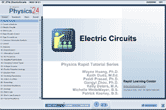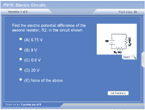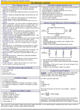How to Learn in 24 Hours?The Rapid Learning Movie

 Need Help? M-F: 9am-5pm(PST): Toll-Free: (877) RAPID-10 US Direct: (714) 692-2900 Int'l: 001-714-692-2900 24/7 Online Technical Support: The Rapid Support Center Secure Online Order:Need Proof? Testimonials by Our Users

 Rapid Learning Courses: MCAT in 24 Hours (2015-16) USMLE in 24 Hours (Boards) Chemistry in 24 Hours Biology in 24 Hours Physics in 24 Hours Mathematics in 24 Hours Psychology in 24 Hours SAT in 24 Hours ACT in 24 Hours AP in 24 Hours CLEP in 24 Hours DAT in 24 Hours (Dental) OAT in 24 Hours (Optometry) PCAT in 24 Hours (Pharmacy) Nursing Entrance Exams Certification in 24 Hours eBook - Survival Kits Audiobooks (MP3)

 Tell-A-Friend: Have friends taking science and math courses too? Tell them about our rapid learning system.Home »  Physics »  College Physics

Electric Circuits

 Topic Review on "Title": Current: Electrical charge flow past a given point per unit of time.  Direct current: Electrical current that flows in only one direction. Alternating current: Electrical current that oscillates forward and backwards. Voltage: The ratio of electric potential energy to electric charge at a particular spot in an electric field.  It is often referred to as voltage since it is measured in volts. Ohm’s law: Basic law that describe current electricity; Voltage equals current times resistance. Internal resistance: Resistance from the processes inside a voltage source; reistance due to the battery itself. Series circuit: A circuit where the components form one continuous loop.  The current is constant throughout. Parallel circuit: A circuit where each component is connected to form its own separate independent branch.  The voltage is constant throughout. Fuse/Circuit breaker: A safety device designed to melt or disconnect a circuit after a predetermined amount of current is exceeded. Junction rule: A restatement of conservation of charge; the current going into a junction must equal the current going out of the junction. Loop rule: A restatement of conservation of energy; the sum of all voltages in the elements of a loop is zero.

Rapid Study Kit for "Title":
 Flash Movie Flash Game Flash Card Core Concept Tutorial Problem Solving Drill Review Cheat Sheet"Title" Tutorial Summary : Electric circuits come in a variety of styles.  Regardless of the type, Ohm’s law can be used to find a few basic quantities.  Voltage is equal to current times resistance.  Also, power can be calculated by current times voltage.  When circuit components are arranged one after another in a loop, this is a series circuit.  When the components are arranged in separate, independent connections to the voltage source, that is a parallel connection.  For more complex circuits, with multiple loops or multiple voltage sources, Kirchoff’s laws are the answer.  The junction rule says that charge is conserved.  The loop rule says that energy or voltage is conserved.

 Tutorial Features: Specific Tutorial Features: • Step by step calculation of complex Kirchoff’s laws problems. • Circuits diagrams simplified into less complex circuits. Series Features: • Concept map showing inter-connections of new concepts in this tutorial and those previously introduced. • Definition slides introduce terms as they are needed. • Visual representation of concepts • Animated examples—worked out step by step • A concise summary is given at the conclusion of the tutorial.

 "Title" Topic List: Ohm’s law Circuit diagrams Alternating current Direct current Internal resistance Electric power Formula Example calculations Series circuits Resistors combined in series Current and voltage characteristics Example calculations Parallel circuit Resistors combined in parallel Current and voltage characteristics Example calculations Kirchoff’s laws Junction rule Loop rule

See all 24 lessons in College Physics, including concept tutorials, problem drills and cheat sheets:
Teach Yourself College Physics Visually in 24 Hours